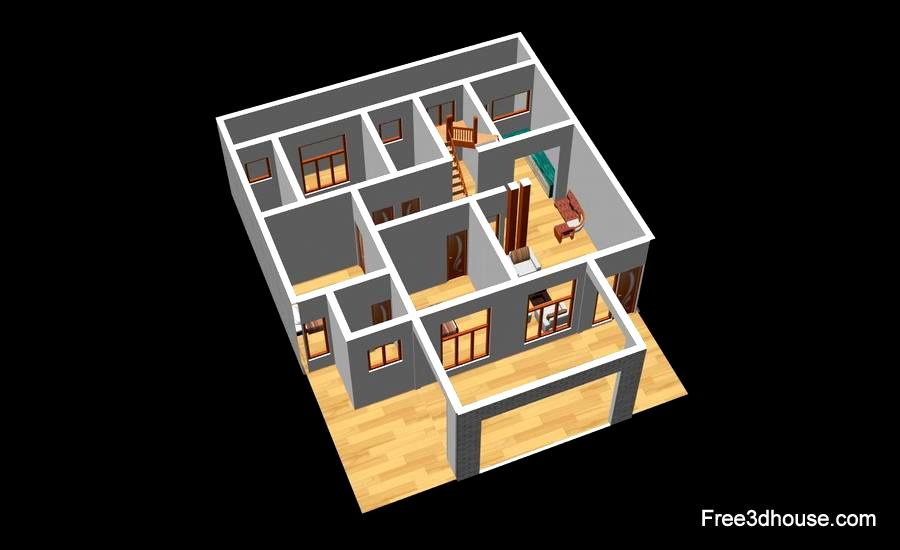# Color image to black and white converter

RGB to grayscale image conversion online:

 Original image:Converted image:

With the Simple Online Image Converter, all you have to do is upload an image and choose the new format that you’re looking for. It is easy, fast and free tool.

## How to convert RGB to grayscale

Gray RGB color code has equal red,green and blue values:

R = G = B

For each image pixel with red, green and blue values of (R,G,B):

R' = G' = B' = (R+G+B) / 3 = 0.333R + 0.333G + 0.333B

This formula can be changed with different weights for each R/G/B value.

R' = G' = B' = 0.2126R + 0.7152G + 0.0722B

Or

R' = G' = B'  = 0.299R + 0.587G + 0.114B

#### Example

Pixel with RGB values of (30,128,255)

The red level R=30.

The green level G=128.

The blue level B=255.

R' = G' = B' = (R+G+B) / 3 = (30+128+255) / 3 = 138

so the pixel will get RGB values of:

(138,138,138)